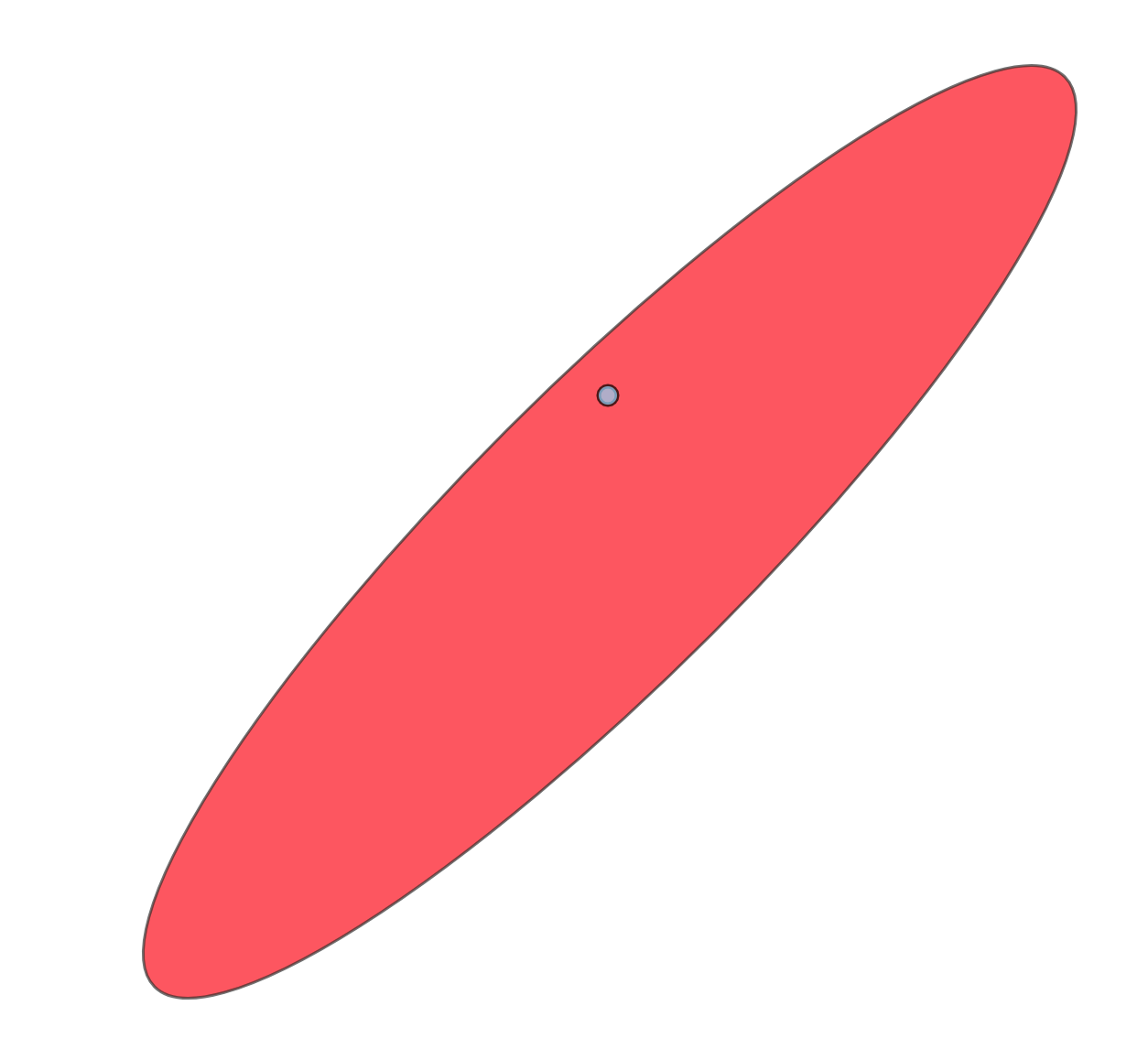# point-in-ellipse

Determine if a point belongs to an ellipse

## Usage no npm install needed!

``````<script type="module">
import pointInEllipse from 'https://cdn.skypack.dev/point-in-ellipse';
</script>``````

# Point in ellipse (Demo)Determine if the point is inside of the ellipse or out, based on ellipse equation.

# Example

``````var inside = require('point-in-ellipse');

var c  = [100, 100];
var rx = 100, ry = 200;
var rotation = 45 * (Math.PI / 180); // rotation in radians

inside(150, 150, c, c, rx, ry, rotation);
// true

``````

# API

``````pointInEllipse(px, py, cx, cy, rx, ry, rotation = 0);
``````

# Usage

CommonJS/Node

``````npm install -S point-in-ellipse;
...
var inside = require('point-in-ellipse');
``````

Browser Simply include the `index.js` file to your project, it will expose global function `pointInEllipse`;